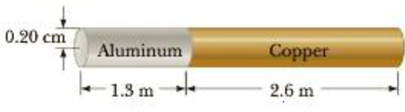Chapter 9, Problem 75P

Chapter
Section
Textbook Problem

Determine the elongation of the rod in Figure P9.75 if it is under a tension of 5.8 × 103 N.Figure P9.75

To determine
The elongation of the rod.

Explanation

Given info: The tension in the rod is 5.8×103N , the radius of the rod is 0.20cm , length of aluminium rod is 1.3m , length of the copper rod is 2.6m , Young’s modulus of aluminium is 7.0×1010Pa , and Young’s modulus of the copper is 11×1010Pa .

Explanation: The total elongation of the rod is the individual elongation of the rods contains aluminium and copper that is ΔLrod=ΔLAl+ΔLCu=(F(L0)Al/AYAl)+(F(L0)Cu/AYCu) . The cross-sectional area of the rod is A=πr2 .

The formula for the elongation of the rod is,

ΔLrod=Fπr2((L0)AlYAl+(L0)CuYCu)

F is the force of tension.

r is radius of the rod.

(L0)Al is length of aluminium.

(L0)Cu is length of copper

Still sussing out bartleby?

Check out a sample textbook solution.

See a sample solution

The Solution to Your Study Problems

Bartleby provides explanations to thousands of textbook problems written by our experts, many with advanced degrees!

Get Started